This example will model and analyze a vivaldi antenna with an internal matching circuit. The vivaldi is also known as an exponentially tapered slot antenna. The antenna possesses wideband characteristics, low cross polarization and a highly directive pattern. The design will be implemented on a single layer dielectric substrate with 2 layers of metal; one for a flared slot line, and the feed line with the matching circuit on the other layer. The substrate is chosen as a low cost FR4 material of thickness 0.8 mm. The design is intended for operation over the frequency band 3.1 - 10.6 GHz .

Antenna Dimensions

The vivaldi antenna is designed to operate between 3 to 11 GHz with dimensions of 4540 mm. At the highest frequency of operation, the structure is approximately. Define the design parameters of the antenna as provided.

Lgnd = 45e-3;
Wgnd = 40e-3;
Ls = 5e-3;
Ltaper = 28.5e-3;
Wtaper = 39.96e-3;
s = 0.4e-3;
d = 5e-3;
Ka = (1/Ltaper)*(log(Wtaper/s)/log(exp(1)));

Create Top Layer Shape

This design consists of three layers; the top layer is the exponentially tapered slot shape. This is the same shape as the vivaldi in the Antenna Toolbox catalog. The bottom layer consists of the feed and the matching circuit. The middle layer is the FR4 substrate. The function pcbStack(ant) converts any 2D or 2.5D antenna from the catalog into a pcb antenna for further modeling and analysis. Create the vivaldi antenna from the catalog and visualize it. Thereafter, move the feed and convert it to the stack representation and access the layer geometry for further modifications.

vivaldiant = vivaldi('TaperLength',Ltaper, 'ApertureWidth', Wtaper,     ...
'OpeningRate', Ka,'SlotLineWidth', s,              ...
'CavityDiameter',d,'CavityToTaperSpacing',Ls,      ...
'GroundPlaneLength', Lgnd, 'GroundPlaneWidth', Wgnd,...
'FeedOffset',-10e-3);
figure
show(vivaldiant);
vivaldiant.FeedOffset = -14e-3;
ewant = pcbStack(vivaldiant);
topLayer = ewant.Layers{1};
figure
show(topLayer)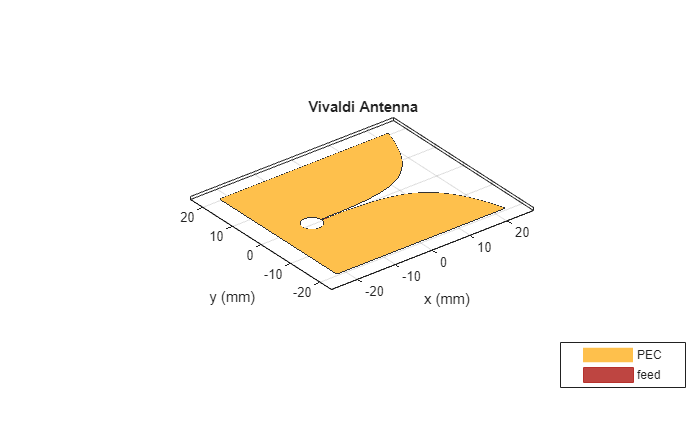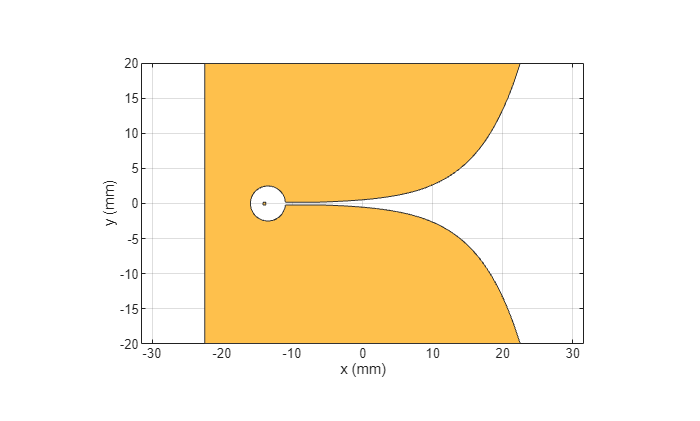Remove Feed Strip from Vivaldi Structure The default vivaldi antenna structure in the catalog has an internal feed and the associated feed strip specified at the center of the antenna. In this example we are using edge feed model. Remove the strip from the vivaldi structure.

cutout = antenna.Rectangle('Length',1e-3,'Width',4e-3,'Center',[-0.014,0]);
topLayer = topLayer-cutout;
figure;
show(topLayer);Create a Matching Circuit for the Vivaldi Antenna

A stepped microstrip line is used as a matching circuit with a 90 degree bend terminating into a radial bowtie stub. Use the rectangle shape primitive in Antenna Toolbox™ to create the stepped microstrip line. Boolean addition operation is used among the shape primitives for this purpose.

L1 = 8e-3;
L2 = 4.1e-3;
L3 = 9.1e-3;
W1 = 1.5e-3;
W2 = 1e-3;
W3 = 0.75e-3;
H = 0.8e-3;
fp = 11.2e-3;
th = 90;
patch1 = antenna.Rectangle('Length',L1,'Width',W1,...
'Center',[-(Lgnd/2 - L1/2), -(Wgnd/2 - fp - W1/2)],...
'NumPoints', [10,2,10,2]);
patch2 = antenna.Rectangle('Length',L2,'Width',W2,...
'Center',[-(Lgnd/2 - L1 - L2/2), -(Wgnd/2 - fp - W1/2)],...
'NumPoints', [5,2,5,2]);
patch3 = antenna.Rectangle('Length',W3,'Width',L3,...
'Center',[-(Lgnd/2 - L1 - L2 - W3/2), -(Wgnd/2 - fp - W1/2 + W2/2- L3/2)],...
'NumPoints', [2,10,2,10]);

Create a Radial Stub Matching circuit To create a radial stub matching circuit we use the function makebowtie. It provides inputs for the radius, neck width, flare angle, center, shape of the bowtie and finally number of points to create a shape for bowtie.

Bowtie = em.internal.makebowtie(8.55e-3, W3, th, [0 0 0],'rounded',20);
rotatedBowtie = em.internal.rotateshape(Bowtie,[0 0 1],[0 0 0],90);
p = antenna.Polygon('Vertices', rotatedBowtie');

radialStub = translate(p, [-(Lgnd/2 - L1 - L2 - W3/2) -(Wgnd/2 - fp - W1/2 + W2/2- L3) 0]);

figure;
show(bottomLayer);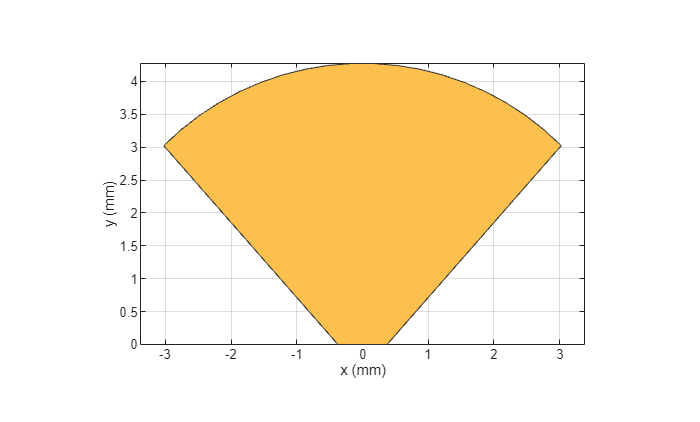Create the PCB stack

Create the board shape for the antenna. The board in this case is rectangular and 45 mm x 40 mm in size.

boardShape = antenna.Rectangle('Length',Lgnd,'Width',Wgnd);
figure;
hold on;
plot(topLayer)
plot(bottomLayer)
grid onDefine the Dielectric Substrate The vivaldi antenna is constructed using an FR4 substrate with relative permittivity of 4.4 and height 0.8 mm.

substrate = dielectric('Name','FR4','EpsilonR', 4.4, 'Thickness', H);

Assign the Layers and Define the Feed Assign the layers starting from the top-layer, in this case vivaldi structure, followed by the FR4 dielectric substrate and finally the lowest layer, which is the matching circuit. The edge-feed is specified between the vivaldi and the matching circuit on the bottom layer. Having the feed line with the matching circuit on the bottom layer reduces any spurious radiation. Define the feed location and the feed diameter as well.

vivaldi_Notch = pcbStack;
vivaldi_Notch.Name = 'vivaldiNotch';
vivaldi_Notch.BoardThickness = H;
vivaldi_Notch.BoardShape = boardShape;
vivaldi_Notch.Layers = {topLayer,substrate,bottomLayer};
vivaldi_Notch.FeedLocations = [-(Lgnd/2), -(Wgnd/2 - fp - W1/2), 1, 3];
vivaldi_Notch.FeedDiameter = W1/2;
figure;
show(vivaldi_Notch);Impedance Analysis

Calculate the antenna impedance over the range of 2.5 GHz to 11 GHz. For the purpose of executing this example, the impedance analysis has been precomputed and save in a MAT-file. The analysis was performed with the mesh generation in the auto mode. Execute the info method on the antenna to get information about the meshing/solution status, analysis frequencies and estimate of the memory required for analysis.

freq = linspace(2.5e9, 11e9,41);
bandfreqs = [3.1e9, 10.6e9];
freqIndx = nan.*(ones(1,numel(bandfreqs)));
for i = 1:numel(bandfreqs)
df = abs(freq-bandfreqs(i));
freqIndx(i) = find(df==min(df));
end
vivaldiInfo = info(vivaldi_Notch)
figure;
impedance(vivaldi_Notch, freq);
vivaldiInfo =

struct with fields:

IsSolved: "true"
IsMeshed: "true"
MeshingMode: "auto"
HasSubstrate: "true"
PortFrequency: [1×41 double]
FieldFrequency: []
MemoryEstimate: "2.2 GB"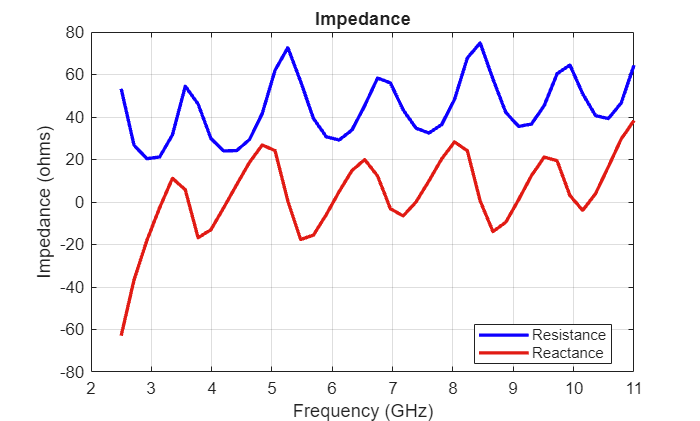Refine the Antenna Mesh

Refine the mesh to check for convergence with the impedance variation over the band. The automatically generated mesh has a maximum edge length of approximately 2 cm and a minimum edge length of 3 mm. The highest frequency in the analysis range is 11 GHz which corresponds to a wavelength in free space of 27.3 mm. Considering 10 elements per wavelength would give us an edge length of approximately 2.7 mm which is lower than the both the maximum and the minimum edge length chosen by the automatic mesher. After a few attempts, using a maximum edgelength of 5 mm and a minimum edge length of 0.8 mm resulted in a good solution.

figure
mesh(vivaldi_Notch, 'MaxEdgeLength',5e-3,'MinEdgeLength',0.8e-3);
view(0,90)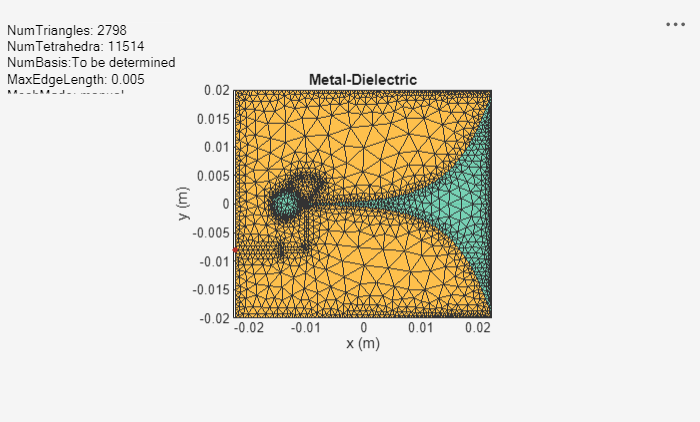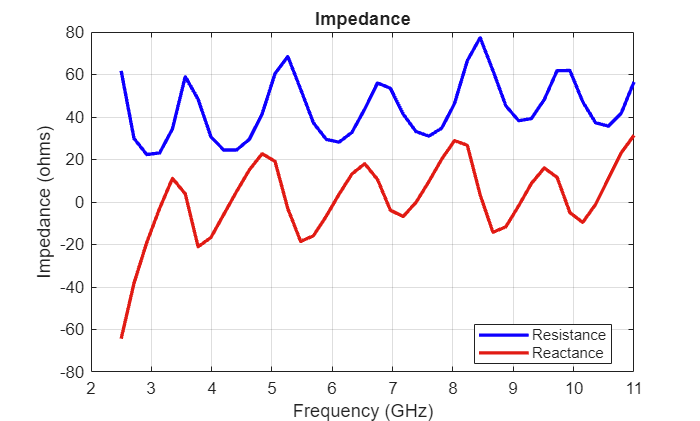Due to the size of the mesh, the number of unknowns increases to obtain an accurate solution. As before the solved structure has been saved to a MAT-file and is loaded here for further analysis.

figure;
impedance(vivaldi_Notch, freq);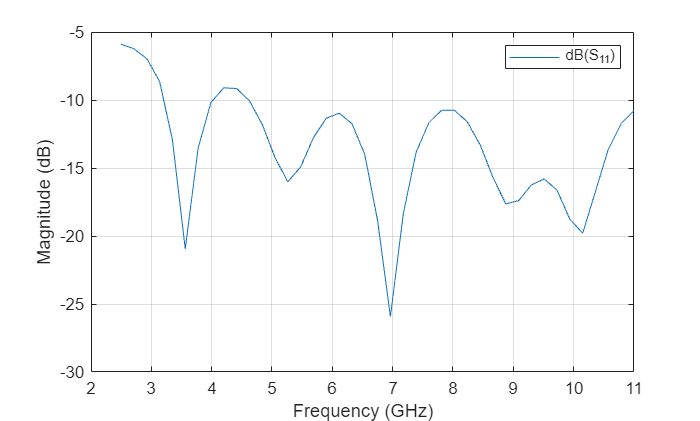Reflection Coefficient

Calculate the reflection coefficient at the input relative to 50-ohm reference impedance. The reflection coefficient is below -10 dB for the frequency range from 3.1 GHz to 11 GHz. Save the reflection coefficient for use later on for calculating the realized gain.

figure;
s = sparameters(vivaldi_Notch, freq);
rfplot(s);
gamma = rfparam(s,1,1);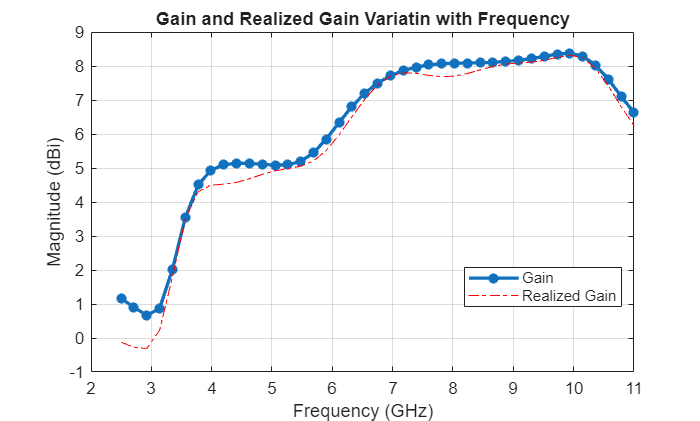Realized Gain

The antenna realized gain includes losses in the dielectric and due to any impedance mismatch. Plot the variation in realized gain with frequency at the antenna boresight at (az,el) = (0,0) deg.

G = zeros(1,numel(freq));
az = 0;
el = 0;
for i = 1:numel(freq)
G(i) = pattern(vivaldi_Notch,freq(i),az,el);
end
g = figure;
plot(freq./1e9,G,'-*','LineWidth',2);
xlabel('Frequency (GHz)');
ylabel('Magnitude (dBi)');
grid on;
title('Gain Variation With Frequency');Compute Mismatch and calculate Realized Gain

mismatchFactor = 10*log10(1 - abs(gamma).^2);
Gr = mismatchFactor.' + G;
figure(g);
hold on
plot(freq./1e9,Gr,'r-.');
legend('Gain','Realized Gain','Location','best')
title('Variation of Gain and Realized Gain with Frequency')
hold offThe wide impedance bandwidth does not necessarily translate to a wide gain/pattern bandwidth. The highest gain is achieved over the 7 - 10.4 GHz range at boresight of approximately 9.5 dBi. Plot the 3D pattern in the middle of this sub-band to understand the overall radiation characteristics.

dfsub = abs(freq - (10.4e9+7e9)/2);
subfreqIndx = find(dfsub==min(dfsub));
figure;
pattern(vivaldi_Notch, freq(subfreqIndx));Phase Center Variation of the Antenna

The phase center of an antenna is the local center of curvature of the far-field phase front . It can vary with frequency and observation angle. An analysis of the phase center variation is critical for positioning systems. This is because variations in the phase center directly translate to variations in time delay, which can impact range estimates between a transmitter and a receiver. To understand this, calculate the maximum possible variation in time delay due to a harmonic signal at f_min and another at f_max over a set of observation angles in the far-field. Choose the angles over 2 orthogonal planes; the first specified at elevation = 0 degrees, i.e. the xy-plane and the other at az = 0 degrees, i.e. xz plane. In the xy-plane we will use thecomponent of the electric field for analysis while in the xz-plane we will use thecomponent of the electric field.

Create Points in Far-Field and Calculate Electric Field Define the far-field sphere radius and the set of observation angles in azimuth and elevation. Choose the two harmonic signal frequencies to be at 3 and 11 GHz respectively.

az = -180:5:180;
el = -90:5:90;
fmin = freq(freqIndx(1));
fmax = freq(freqIndx(2));
R = 100*299792458/fmin;
coord = 'sph';
phi = 0;
theta = 90 - el;
[Points, ~, ~] = em.internal.calcpointsinspace( phi, theta, R,coord);

Calculate Local Phase Variation of E-field Find the electric field at the two frequencies and convert to the spherical component. Since we are interested in the maximum time delay variation, we first calculate the maximum phase variation between the two frequencies over the set of points in XZ-plane.

E_at_fmin = EHfields(vivaldi_Notch,fmin,Points);
E_at_fmax = EHfields(vivaldi_Notch,fmax,Points);
Eth_at_fmin = helperFieldInSphericalCoordinates(E_at_fmin,phi,theta);
Eth_at_fmax = helperFieldInSphericalCoordinates(E_at_fmax,phi,theta);
phase_at_fmin = angle(Eth_at_fmin);
phase_at_fmax = angle(Eth_at_fmax);

Calculate Time Delay Variation Between the Two Harmonic Signals

delta_phase = max(phase_at_fmin-phase_at_fmax) - min(phase_at_fmin-phase_at_fmax);
delta_omega = 2*pi*(fmax-fmin);
delta_time = pi*delta_phase/180/delta_omega;
delta_timeXZ = delta_time*1e12;
sprintf("The time delay variation in the XZ-plane is: %2.2f %s",delta_timeXZ,'ps')
ans =

"The time delay variation in the XZ-plane is: 2.18 ps"

Repeat the process for the points on the XY-plane and calculate the time delay variation due to thevariation.

phi = az;
theta = 0;
delta_omega = 2*pi*(fmax-fmin);
[Points, ~, ~] = em.internal.calcpointsinspace( phi, theta, R,coord);
E_at_fmin = EHfields(vivaldi_Notch,fmin,Points);
E_at_fmax = EHfields(vivaldi_Notch,fmax,Points);
[~,Ephi_at_fmin] = helperFieldInSphericalCoordinates(E_at_fmin,phi,theta);
[~,Ephi_at_fmax] = helperFieldInSphericalCoordinates(E_at_fmax,phi,theta);
phase_at_fmin = angle(Ephi_at_fmin);
phase_at_fmax = angle(Ephi_at_fmax);
delta_phase = max(phase_at_fmin-phase_at_fmax) - min(phase_at_fmin-phase_at_fmax);
delta_time = pi*delta_phase/180/delta_omega;
delta_timeXY = delta_time*1e12;
sprintf("The time delay variation in the XY-plane is: %2.2f %s",delta_timeXY,'ps')
ans =

"The time delay variation in the XY-plane is: 2.71 ps"

Observation The time delay variation in the two planes that bisect the antenna boresight, reveal that the phase center is relatively stable. The average time delay variation of approximately 2 ps translates to a maximum range error of less than 1 mm.

Generate Gerber Files for Prototyping

The vivaldi antenna can be fabricated by using the gerber file generation capability in the toolbox. For this example and SMA Edge connector from Amphenol  was chosen and Advanced Circuits  was used as the manufacturing service. In addition, on the PCBWriter object, we chose to not enable the layer of solder mask. The fabricated antenna, is shown below.Performance in the 3-6 GHz Range

The fabricated antenna was tested using a desktop network analyzer. Since the upper limit of the analyzer was 6.5 GHz we compare the results of the antenna with the analysis from the model.

fLim = 6.5e9;
findx = find(freq>fLim);
freq2 = freq(1:findx(1)-1);
s_model = sparameters(vivaldi_Notch, freq2);
rfplot(s_model);
s_proto = sparameters('UWB2.s1p');
hold on
rfplot(s_proto)
legend('Model','Measured','Location','best')Conclusion

The proposed antenna covers the Federal Communications Commission defined UWB spectrum and has more than 3.5:1 impedance bandwidth (from 3 GHz to more than 11 GHz). The antenna realized gain achieved over the band 3-10 GHz at boresight is very close to the gain result. Comparing the reflection coefficient in 3 - 6 GHz range of the fabricated prototype and the model reveals an acceptable performance. The reflection coefficient between 4-4.75 GHz does degrade to about -8 dB.

Reference

. High Gain Vivaldi Antenna for Radar and Microwave Imaging Applications International Journal of Signal Processing Systems Vol. 3, No. 1, June 2015 G. K. Pandey, H. S. Singh, P. K. Bharti, A. Pandey, and M. K. Meshram.

. Vishwanath Iyer, Andrew Cavanaugh, Sergey Makarov, R. J. Duckworth,'Self-Supporting Coaxial Antenna with an Integrated Balun and a Linear Array Thereof', Proceedings of the Antenna Application Symposium, Allerton Park, Monticello, IL, pp.282-284, Sep. 21-23rd 2010.